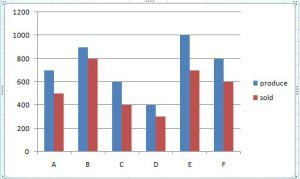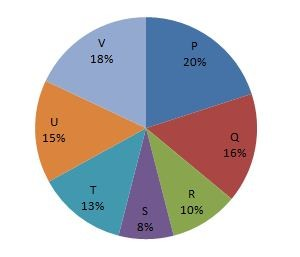#Quants Questions : Data Interpretation Set 10

Hello Aspirants. Welcome to Online Quantitative Aptitude section in AffairsCloud.com. Here we are creating question sample From Data Intrepretation that is important for IBPS,SBI exam,SSC exam, Railways Exam,FCI exam  and other competitive exams. We have included Some questions that are repeatedly asked in exams !!

I.Direction(1-5): Study the following graph carefully to answer the questions given     belowP : 700  900  600  400  1000  800
S:  500  800  400  300  700   600
A       B     C       D     E        F

1. What is the average number of units produce by all companies together ?(approx)
A)700
B)720
C)733
D)740
E)750
Explanation :
Avg production = (700+900+600+400+1000+800)/6 = 733.33 = 733

2. Which company had the lowest percentage of sales with respect to its production ?
A)Company A
B)Company B
C)Company C
D)Company D
E)Company E
Explanation :
A = (500×100)/700 = 71.42%
B = (800×100)/900 = 88.88%
C = (400×100)/600 = 66.66%
D = (300×100)/400 = 75%
E = (700×100)/1000 = 70.%
F = (600×100)/800 = 75%

3. What is the ratio of the total production of the company B and C together to the total sales of the same companies ?
A)4:5
B)5:4
C)5:2
D)2:3
E)None of these
Explanation :
P = 900+600 = 1500
S = 800+400 = 1200
P : S = 1500 : `1200 = 5:4

4. The total unit sold by  D ,E ,F together is approximately what percentage of the total units produced by these companies ?
A)72%
B)72.12%
C)72.22%
D)72.72%
E)73.67%
Explanation :
S = 300+700+600 = 1600
P = 400+1000+800 = 2200
S/P = (1600×100)/2200 = 72.72%

5. What is the ratio of total production of all the companies to the total sales of all the companies ?
A)4:3
B)3:4
C)3:2
D)2:3
E)None of these
Explanation :
P = 700+900+600+400+1000+800 = 4400
S = 500+800+400+300+700+600 = 3300
P:S = 4400 : 3300 = 4: 3

II. Direction (1-5) : Refer to the pie-chart and answer the given questions.
Distribution of the total number of novelssold by 7 stores.Total number of novels= 6300.1. What is the ratio of the number  of novels sold by store R to the total number of novels sold by store P and S ?
A)5:4
B)5:14
C)14:5
D)4:5
E)None of these
Explanation :
R = (6300×10)/100 = 630
P & S = (6300×28)/100 = 1764
630 : 1764 => 5:14

2. What is the average number of novels sold by store P, Q and R
A)960
B)962
C)964
D)956
E)966
Explanation :
P = (20×6300)/100 = 1260
Q = (16×6300)/100 = 1008
R = (10×6300)/100 = 630
Avg = (1260+1008+630)/3 = 966

3. Number of novels sold by R is what percentage of the number of novels sold by P and Q ?
A)27.76
B)27.77
C)27.78
D)27.79
E)None of these
Explanation :
P = 1260
Q = 1008
R = 630
% = [630/(1260+1008)]×100 = 27.77

4. What is the total number of novels sold by store T, U and V ?
A)1860
B)1861
C)1862
D)1863
E)None of these
Explanation :
T = (13×6300)/100 = 819
U = (15×6300)/100 = 945
V = (18×6300)/100 = 1134
Total = 819 + 945 + 1134 = 2898

5. What is the difference between Number of novels sold by V to  number of sold by R ,S and T store ?
A)810
B)813
C)819
D)836
E)None of these